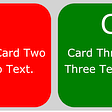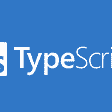# Introduction to Functors in Typescript

## Let me tell you what functor is right now for OOP : It’s a container. together with a mapping function.

Functor are the base of category theory which have become one of the most valuable tools of functional programming paradigm. Also Typescript is becoming more and more functional. Typescript developers should be familiar with the functional paradigm.

In mathematics, a functor is a map between categories. A category is just a collection of objects and arrows between objects like below. Category c has the objects x,y and an arrow f from x to y. That’s all there is to it basically. kind of.

In Programming one major category is this of Types. The arrows between the types are the functions/methods. But because the concept is so broad, categories arise in all kind of areas inside programming. For example If we get the category where objects are programs then the arrows between them could be Compilers, that get an input program and give a different program.

Ok, lets get back to Functors. A functor F just maps objects from C, to an other category D.We will start by looking at the minimum structure that qualifies as a functor in TS:

This is the minimum construction that we could call a functor because it has exactly two things

1. A “constructor” that lifts an object T to `Id<T>`

2. and it has a mapping method `map<T1>(f: (y: T) => T1) `that lifts functions f

`class Id<T>{    Value: T     constructor(value: T) {              //requirement (1)        this.Value = value;                  }     map<T1>(f: (y: T) => T1): Id<T1> {   //requirement (2)        return new Id<T1>(f(this.Value))     }; }`

Because it’s the minimal functor structure it goes by the name Identity functor. Let us see a simple example where we have two integers 2 and 4 (here we take for simplicity the category of integers as our initial category C) also in this category there is the function `square = x=>x*x` that maps 2 to 4.

If we apply the Id(_) constructor we can map each integers to the Id[int] category. For example 2 will be mapped to Id(2) and 4 maps to Id(4), the only part missing is the correct lifting of the function f Id[f] to this new category. It’s easy to see that the correct mapping is:

`map<T1>(f: (y: T) => T1): Id<T1> {return new Id<T1>(f(this.Value))};becausevar square  = x=>x*x;new  Id(2).map(square) === new Id(square(2))`

# Commutative Diagrams

There is one important thing about the mapping function, though. The mapping should get the same result for  if we take any of the two possible routes to get there. This means map (aka lifting of a function from C to D) should preserve the structure of C.

1. We could first get the Functor and then map it. This is the red path on the diagram.

`Id(y) = Id(x).map(f);`

2. Or first lift 2 with f and then get the Functor.

`Id (y) = Id (f(x));`

Two objects that were connected with an arrow in category C, should also be connected with an arrow in category D. And reversely, if there was no connection in C there should be no connection in D.

When the red and blue paths give the same result, then we say that the diagram commutes

Moreover, that means that the lifting of morphisms (aka arrows, aka functions in programming) preserves the structure of the objects in C.

In practical day to day programming, commutating diagrams means that we can exchange the order of operations and still get the same result. It is not something that happens automatically and is something very helpful to have when coding

# Extending Promise<T> to Functor

In the following sections, we will see some examples of other popular Functors in TS. We will start by extending Promise, by providing a map method that obeys the functor laws and thus promote native Promise into a functor.

We have said that the usual metaphor for a functor is “a container.” A Promise can be seen as a container that takes a value and wraps it, until it is resolved. In order to promote Promise to Functor, there must be a mapping function that would be able to lift any function and give a new Promise with the lifted value.

Here is one possible mapping function that preserves structure:

The mapping function that lifts the function f: T → T1 It follows the steps:

1. Waits for the result of the Promise (so in a way unwraps the contained value)` this.then(x => {…})`

2. Applying the function `f(x)`

3. wraps the resulting value again into a new Promise: `this.then(x => resolve(f(x)))`

because when we implement a map, we always return something of the same type in order to belong to the same category (in this case type) or Promise.

Excerpt from the book :

--

--

--

More than 14 years’ experience as full stack developer and Software Architect .https://leanpub.com/u/dimitrispapadim

Love podcasts or audiobooks? Learn on the go with our new app.

## Automate boring files organizing using python (Python files organizer)## Mutual TLS made easy with OpenShift Service Mesh, Part 2## Preparation guide on CKS( Certified Kubernetes Security Specialist)## Development Update — May, 2022## Responsive CSS for Mobile View without Media QueryMore than 14 years’ experience as full stack developer and Software Architect .https://leanpub.com/u/dimitrispapadim

## Retry Async Await in Typescript with TypeGuard## Basic TypeScript usage (part 1)## How to Use the Union Operator to Create Mixed Type Arrays in TypeScript